Function: flatten - entries of a structure involving lists, sets, or arrays

Calling sequence:
flatten(expr);

Parameters:

expr - the expression

Description:

This function takes a structure composed of sets, lists, arrays and/or tables, and returns its entries as a sequence (an entry in this case being anything other than a set, list, array or table).

The entries of an array or table are returned in order of the indices, using numerical order for numerical indices and alphabetical order for indices that are names. The entries of a list or set are returned in their order within the list or set (note that the order of elements in a set is arbitrary, but once the set exists in Maple its order will not change within the session).

This function is part of the Maple Advisor Database library, and must be loaded before use by the command readlib(flatten); .

Examples:

> flatten([{{a,b},{c,d}}]);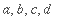> M:= matrix([[1,2],[3,2]]);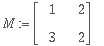> flatten(M);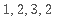> Q:= array(1..2,1..2,1..2,[[[1,2],[3,4]],[[5,6],[7,8]]]):

> flatten(Q);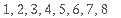In the following example, T is implicitly constructed as a table by assigning values to its elements. Note that convert(T, list) does not return the entries in the order of the indices, but flatten(T) does.

> T:= first: T:= third: T:= second:

> convert(T,list);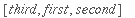> flatten(T);